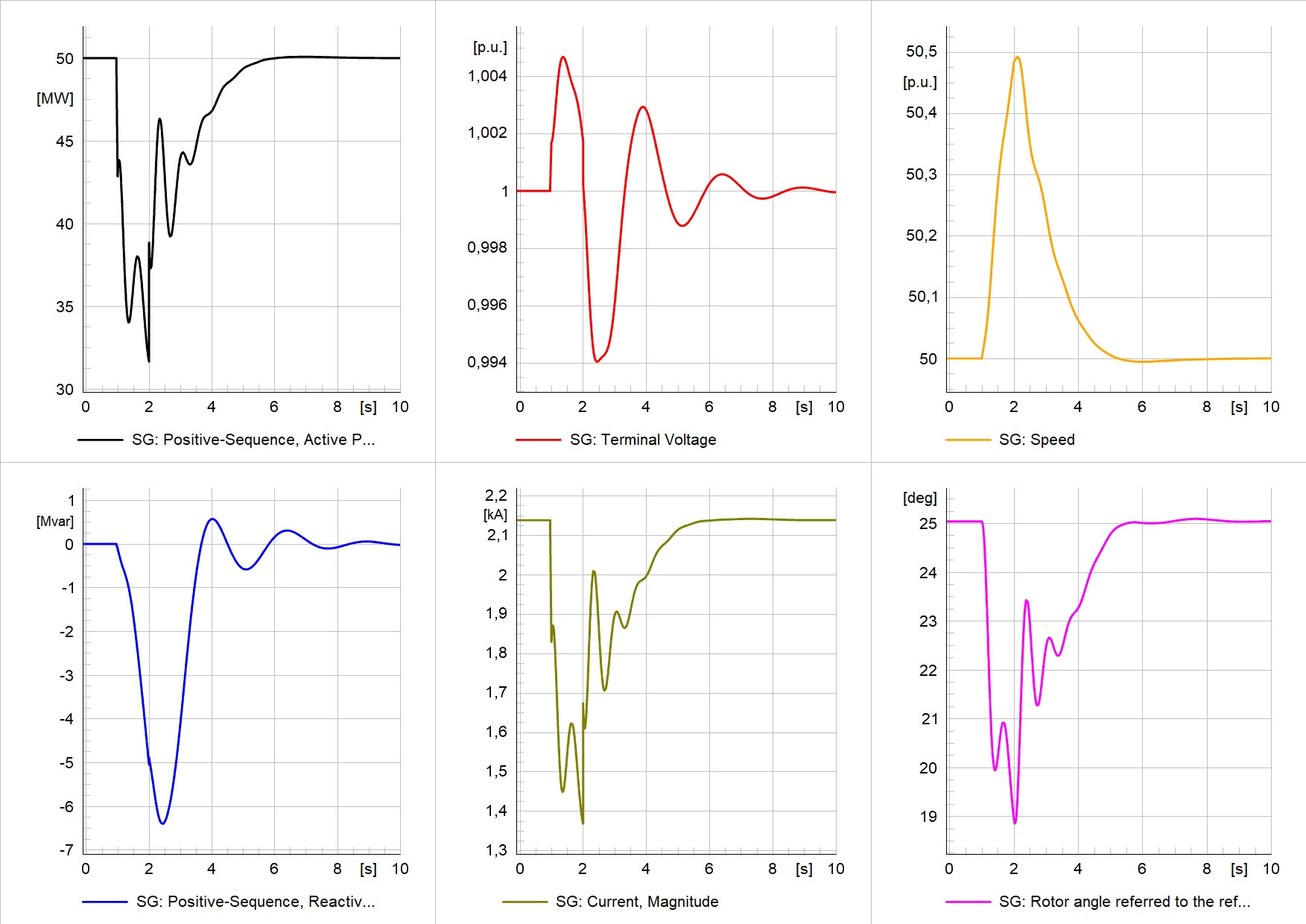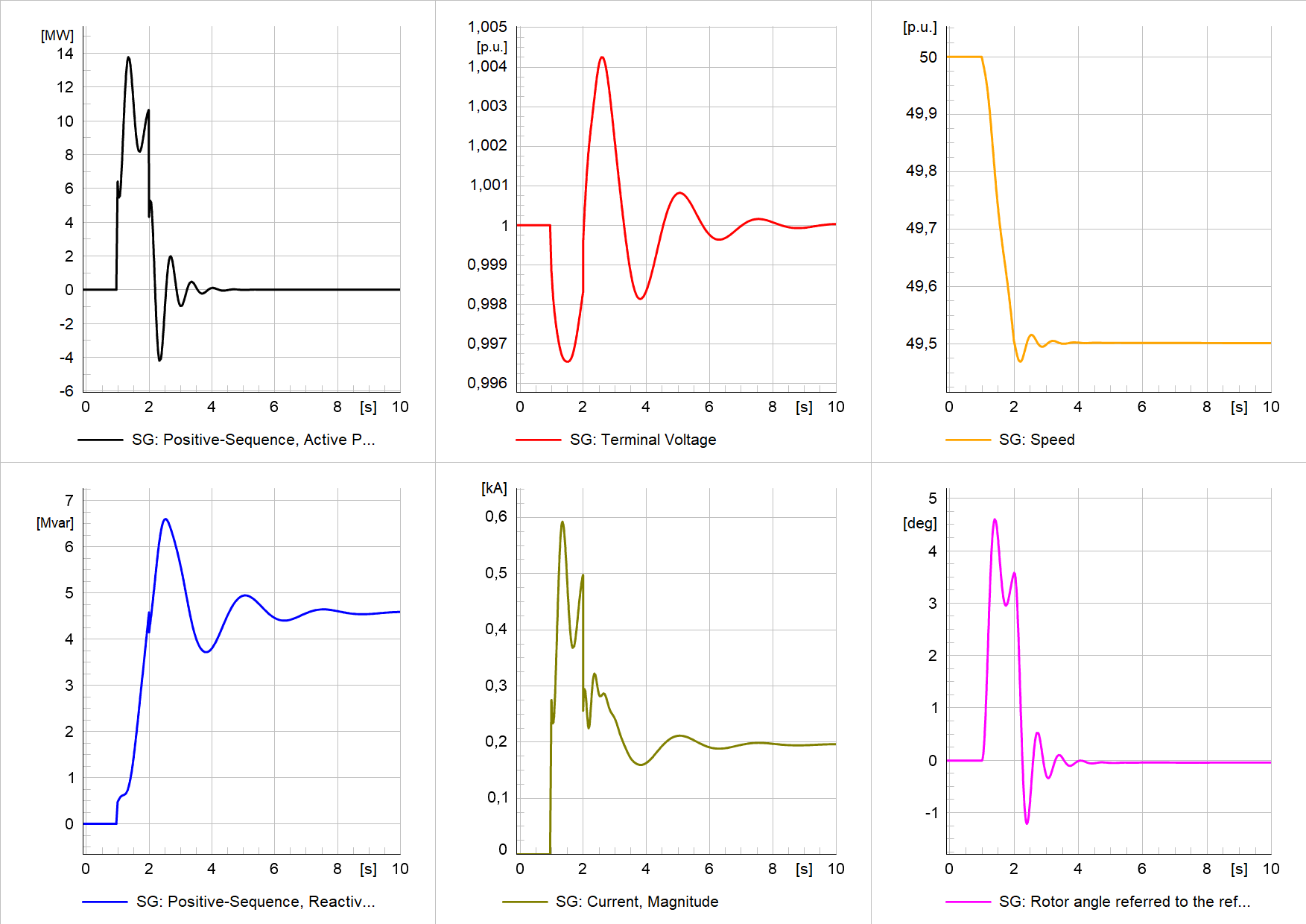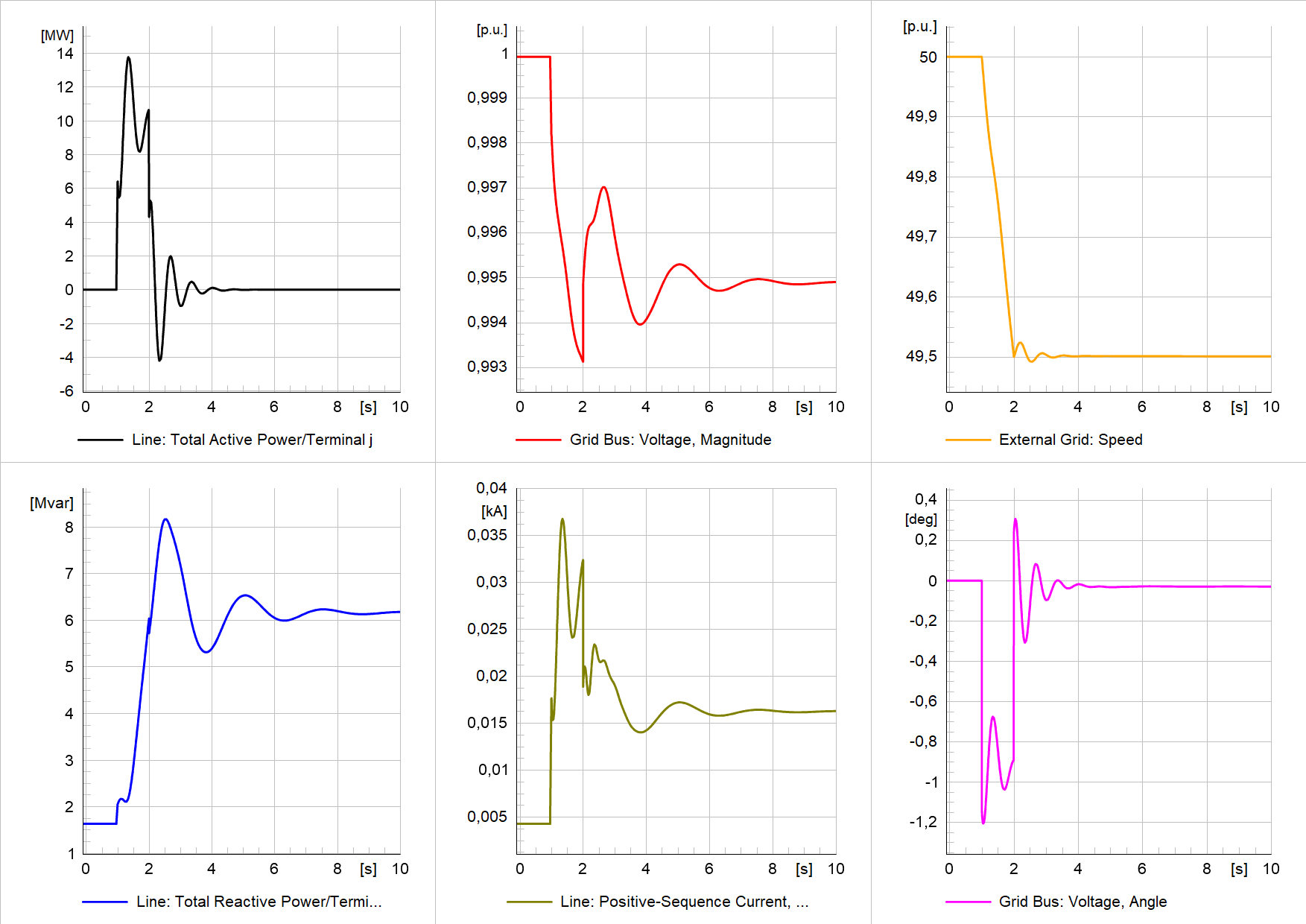# A template for rate-of-change-of-frequency (RoCoF) analysis of synchronous generators/condensers

The importance of grid connection studies increases around the world. TSOs focus more on the grid impact of new generation units as the energy transition moves forward. Adding more converter-based units changes the power system dynamics fundamentally, e.g. higher frequency gradients. That’s also why TSOs require rate-of-change-of-frequency (RoCoF) analyses before the approval of prospective units.

Similarly important is the fault-ride-through (FRT) behavior. Check this post for more information: A template for fault-ride-through (FRT) analysis of synchronous generators/condensers

Here, I provide a template for RoCoF analysis of synchronous generators including a step-by-step guide on how to use it. It is also suitable for synchronous condensers because they are like synchronous generators, just without a prime mover. The template is implemented in DIgSILENT PowerFactory.

## Single-machine infinite-bus (SMIB) concept

The PowerFactory model includes a single-machine infinite-bus model. The model consists of a synchronous generator, transformer, line/cable, load, and the external grid as shown below.

## Synchronous generator excitation and voltage control

To model the excitation and voltage control of the synchronous generator, I used the IEEE ST3C model. This is a purely arbitrary choice as the model needs to be adapted according to the investigated generator’s real excitation and voltage control system.

## Synchronous generator turbine and governor

To model the turbine and governor of the synchronous generator, I used a simplified gas turbine and governor model (GAST – acc. to IEEE PES-TR1). This is a purely arbitrary choice as the model needs to be adapted according to the investigated generator’s real turbine and governor systems.

## How to achieve the desired RoCoF?

Now the question is how to achieve the desired RoCoF in the simulation? To answer this, we need to go back to the basics and remember why the frequency of a power system changes.

The frequency of a power system is a measure of the active power balance, i.e. whenever there is an imbalance between supply and demand, the frequency changes. If there is a surplus of supply, the frequency increases, and vice versa.

Knowing that we see two possibilities to introduce a RoCoF. Either we change the supply or the demand. Since we want to analyze a synchronous generator under certain conditions, it makes sense to introduce the RoCoF through a change in the demand of the system.

Therefore, I have added a load at the “Grid Bus” (please refer to the screenshot of the grid). This load is used to introduce a power imbalance that leads to the desired RoCoF as follows:

1. Initially, the load is disconnected (breaker open).
2. During the simulation, the load is being connected (by closing the breaker).
3. The RoCoF depends on the size of the load. The direction depends on the sign of the load (positive active power = load, negative active power = generation)
4. Finally, the load is disconnected again after a specific time (by opening the breaker).

The next question is, how big do I need to set the load to achieve the desired RoCoF? This, in turn, depends on quite a number of parameters, e.g. the inertia of the synchronous generator and the external grid. That is why I prepared a Python script to determine the active power setpoint given the RoCoF and target frequency. The RoCoF and target frequency can be selected by the user as shown below. The Python script is included in the download.

## Study cases for the RoCoF analysis

Generally, grid connection studies assess the impact of the unit at the Point of Connection (PoC). The following study cases represent a selection of scenarios that could be of interest.

The following four study cases are presented:

### Study case 1 – negative RoCoF with governor (generator mode)

The frequency starts at the nominal frequency, decreases at the specified RoCoF until the target frequency. Here, the RoCoF event lasts for 1 s (depends on RoCoF and target frequency). The table below summarizes the simulation setup.

The most important results are the generator variables and the variables at the PoC. The plot below shows the results for the generator variables: active/reactive power, terminal voltage, generator current, speed, and rotor angle.

Since the synchronous generator is equipped with a GOV model with droop control considering the frequency deviation, after the RoCoF event, the frequency recovers to the nominal value.Simulation results of study case 1: Generator variables –active/reactive power, terminal voltage, generator current, speed, and rotor angle

In the plot below you can find the results for the PoC. Here it is important that the branch variables active/reactive power and current represent the contribution from the generator (through the connected line). The voltage magnitude and angle are bus variables and given for the PoC (which I call Grid Bus here). The speed of the external grid represents the frequency (which is also used in the Python script to determine the corresponding active power of the load).Simulation results of study case 1 at PoC –active/reactive power and current (contribution from the unit); voltage magnitude, voltage angle, and speed (frequency) at the PoC

### Study case 2 – positive RoCoF with governor (generator mode)

The frequency starts at the nominal frequency, increases at the specified RoCoF until the target frequency. Here, the RoCoF event lasts for 1 s (depends on RoCoF and target frequency). The table below summarizes the simulation setup.

The most important results are the generator variables and the variables at the PoC. The plot below shows the results for the generator variables: active/reactive power, terminal voltage, generator current, speed, and rotor angle.

Since the synchronous generator is equipped with a GOV model with droop control considering the frequency deviation, after the RoCoF event, the frequency recovers to the nominal value.Simulation results of study case 2: Generator variables –active/reactive power, terminal voltage, generator current, speed, and rotor angle

In the plot below you can find the results for the PoC. Here it is important that the branch variables active/reactive power and current represent the contribution from the generator (through the connected line). The voltage magnitude and angle are bus variables and given for the PoC (which I call Grid Bus here). The speed of the external grid represents the frequency (which is also used in the Python script to determine the corresponding active power of the load).Simulation results of study case 2 at PoC –active/reactive power and current (contribution from the unit); voltage magnitude, voltage angle, and speed (frequency) at the PoC

### Study case 3 – negative RoCoF w/o governor (condenser mode)

The frequency starts at the nominal frequency, decreases at the specified RoCoF until the target frequency. Here, the RoCoF event lasts for 1 s (depends on RoCoF and target frequency). The table below summarizes the simulation setup.

The most important results are the generator variables and the variables at the PoC. The plot below shows the results for the generator variables: active/reactive power, terminal voltage, generator current, speed, and rotor angle.

Since we are operating in synchronous condenser mode (i.e. no prime mover), the active power of the unit is equal to zero before the RoCoF event. During the event active power is released to the grid from the energy stored in the rotating mass. However, as there is no governor to control the turbine power (to recover the released energy) the frequency stabilizes at a lower value after the RoCoF event.Simulation results of study case 3: Generator variables –active/reactive power, terminal voltage, generator current, speed, and rotor angle

In the plot below you can find the results for the PoC. Here it is important that the branch variables active/reactive power and current represent the contribution from the synchronous condenser (through the connected line). The voltage magnitude and angle are bus variables and given for the PoC (which I call Grid Bus here). The speed of the external grid represents the frequency (which is also used in the Python script to determine the corresponding active power of the load).Simulation results of study case 3 at PoC –active/reactive power and current (contribution from the unit); voltage magnitude, voltage angle, and speed (frequency) at the PoC

### Study case 4 – positive RoCoF w/o governor (condenser mode)

The frequency starts at the nominal frequency, increases at the specified RoCoF until the target frequency. Here, the RoCoF event lasts for 1 s (depends on RoCoF and target frequency). The table below summarizes the simulation setup.

The most important results are the generator variables and the variables at the PoC. The plot below shows the results for the generator variables: active/reactive power, terminal voltage, generator current, speed, and rotor angle.

Since we are operating in synchronous condenser mode (i.e. no prime mover), the active power of the unit is equal to zero. That also means that there is no governor to control the turbine power and, thus, the frequency stabilizes at a higher value after the RoCoF event.

Since we are operating in synchronous condenser mode (i.e. no prime mover), the active power of the unit is equal to zero before the RoCoF event. During the event active power is drawn from the grid and the energy is stored in the rotating mass. However, as there is no governor to control the turbine power (to release the drawn energy) the frequency stabilizes at a higher value after the RoCoF event.Simulation results of study case 4: Generator variables –active/reactive power, terminal voltage, generator current, speed, and rotor angle

In the plot below you can find the results for the PoC. Here it is important that the branch variables active/reactive power and current represent the contribution from the synchronous condenser (through the connected line). The voltage magnitude and angle are bus variables and given for the PoC (which I call Grid Bus here). The speed of the external grid represents the frequency (which is also used in the Python script to determine the corresponding active power of the load).Simulation results of study case 4 at PoC –active/reactive power and current (contribution from the unit); voltage magnitude, voltage angle, and speed (frequency) at the PoC

## A step-by-step guide to carry out the RoCoF analysis

In the following, I provide a step-by-step guide on how to do a RoCoF analysis on your own.

2. Change the parameters of the equipment
• Generator
• Transformer
• Line
• External Grid. Note: when analyzing RoCoF events, I highly recommend to set the acceleration time constant of the external grid to a reasonable value of the real grid instead of a high value (which is often used to represent an infinite bus). Why? Because otherwise you need a very large load to achieve the desired RoCoF as the stored energy in the rotating mass of the external grid increases linearly with the inertia. This might lead to convergence issues in the load flow calculation.
3. If necessary, change the voltages of the buses (SG Bus, HV Bus, Grid Bus).
4. Change the common model of the excitation and AVR and adapt the parameters to comply with your equipment
5. Change the common model of the turbine-governor system and adapt the parameters to comply with your equipment
6. Set the active and reactive power of the synchronous generator as desired
7. Set the voltage setpoint at the synchronous generator terminal through the station control (the external grid controls the initial voltage at the synchronous generator terminal)
8. Open the Python script and define:
• Path to your PowerFactory installation (line 32 in the script)
• project name
• study case
• target RoCoF and frequency
• starting time of the RoCoF event
9. Run the Python script (don’t forget to close PowerFactory, otherwise you can’t run the script)
10. Open PowerFactory and analyze the results (restart Python console before opening PowerFactory to avoid license problems)

I hope you enjoyed this article. If so, you might also like the following: A template for fault-ride-through (FRT) analysis of synchronous generators/condensers

More insights to follow in the next article.

Michael